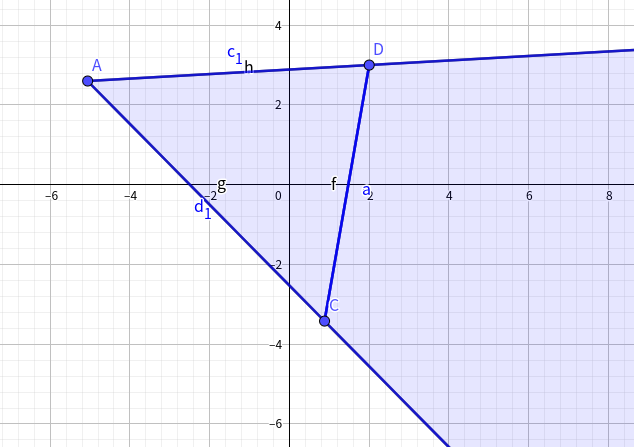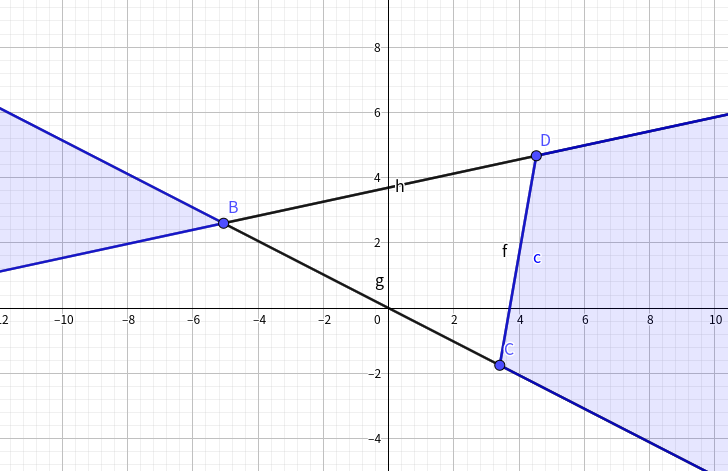# JOISC部分题解

code

code

code

code

code

code

## 2015

### Keys

$S,S:$前面的人带钥匙有时间间隔的贡献
$T,S:$直接给答案加上时间间隔的贡献
$T,T:$后面的人带钥匙有时间间隔的贡献
$S,T:$两人同时带钥匙有时间间隔的贡献

code

### Card Game Is Great Fun

$f_{i,j,k}$表示第$3$张牌是$i$，第$1$张牌是$j$，第$2$张牌是$k$，上次选出的牌是$i-1$的最大贡献，设$g_{i,j,k}$表示第$3$张牌是$i$，第$1$张牌是$j$，第$2$张牌是$i-1$，上次选出的牌是$k$的最大贡献。

code

upd on 2021.1.20：

$\begin{cases} f_{i,j,k}\to f_{i+1,j,k}\\ g_{i,j,k}\to f_{i+1,j,i}\\ f_{i,j,k}\to g_{i+1,k,j}\\ g_{i,j,k}\to g_{i+1,i-1,j} \end{cases}$

$f_{i,j,k}\to f_{i+1,j,k}:$ 条件是 $i-1$ 后可以直接跟 $i$，直接继承。
$g_{i,j,k}\to f_{i+1,j,k}:$ 条件是 $k$ 后可以直接跟 $i$，枚举 $j$ 用先前预处理的 $\text{bitset}$ 判断。
$f_{i,j,k}\to g_{i+1,k,j}:$ 条件是 $i-1$ 后可以直接跟 $j$，枚举 $j$ 后和之前预处理的 $\text{bitset}$ 进行 $\text{AND}$ 操作即可。
$f_{i,j,k}\to g_{i+1,i-1,j}:$ 条件是 $k$ 后可以直接跟 $j$，和第二种情况一样的枚举 $j$$\text{bitset}$ 判断。

code

code

code

## 2016

### 棋盘游戏

f_{i,j,0}= \begin{aligned} \begin{cases} \sum f_{i-1,k,0}\times {j-1\choose cnt}cnt!\\ \sum f_{i-1,k,1}\times {j-1\choose cnt}cnt!,\ \ k+cnt\geq j \end{cases} \end{aligned} \\ f_{i,j,1}= \begin{aligned} \begin{cases} \sum_{k<j} f_{i-1,k,0} \times cnt!\times({siz-1\choose cnt}-{j-1\choose cnt}),k<j-1\\ \sum_{k<j} f_{i-1,k,0} \times cnt!\times {siz-j\choose cnt},k=j-1 \end{cases} \end{aligned}

code

### 三明治

$O(n^4)$直接枚举某个位置然后记搜求出答案。
$O(n^3)$的话，可以发现删除某个位置必须删除其左边到右边的所有位置，可以对于某一行从左往右删一下，再从右往左删一下，就是$O(n^3)$的了。
code

code

code

code

## 2017

code

code

code

code

### 长途巴士

\begin{aligned} \begin{cases} f_i = f_{i-1} + \lceil \frac{X - D_i}{T} \rceil \times W\\ f_i = \min\limits_{0 \leq j < i} f_j + (i - j) \times W \times cnt + \sum\limits_{k = j + 1}^i C_k \end{cases} \end{aligned}

$\sum C$拆成前缀和然后斜率优化单调栈维护凸壳即可。
code

code

code

### Dragon 2code

posted @ 2020-11-30 21:09  heyujun  阅读(605)  评论(2编辑  收藏  举报